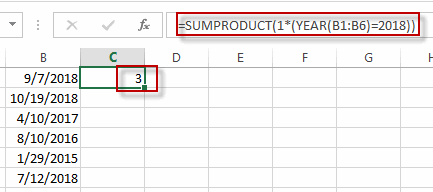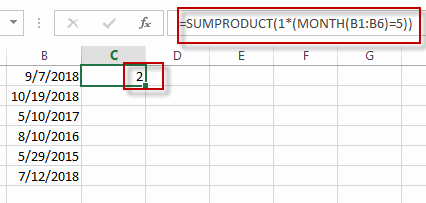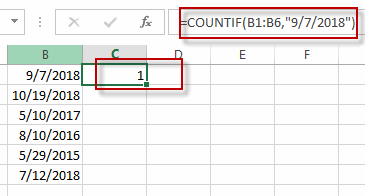# Count Dates in Given Year/Month/Day in Excel

This post will guide you how to count dates in a give year or month or day with a formula in Excel. How do I count cells based on year, month or day in Excel. How to countif by a specified date (Year or month) in a range of cells in Excel.

## Count Dates in Given Year

Assuming that you have a list of data in range B1:B6 that contain date values, and you want to count those dates by a specified year(2018), how to achieve it. or you want to count the dates by a specified month (5).

You can create a formula based on the SUMPRODUCT function and the YEAR function to count dates by a give year. Just like this:

`=SUMPRODUCT(1*(YEAR(B1:B6)=2018))`

Type this formula into a blank cell and then press Enter key to apply this formula.

###The count number is calculated by this formula in Cell C1.

You can also use another excel array formula based on the SUM function , the IF function and the YEAR function to achieve the same result.

`=SUM(IF(YEAR(B1:B6)=2018,1))`

The Year function will convert the data value to a year value only. and the IF function will check if the year value returned by the YEAR function is equal to the given year, if so, return value 1. otherwise, returns nothing. and the sum function will sum the array list that returned by the IF function.

You need to type this formula into a cell, and then press Ctrl + Shift + Enter keys in your keyboard to convert this formula as a array formula.

## Count Dates in a given Month

To count dates in a given month, you can create a formula based on the SUMPRODUCT function and the Month function. For example, you want to count datas in range of cells B1:B6 by a given month value 5. you can write down this formula:

`=SUMPRODUCT(1*(MONTH(B1:B6)=5))`

###Or you can also write down an excel array formula based on the SUM function, the IF function and the MONTH function. Just like this:

`=SUM(IF(MONTH(B1:B6)=5,1))`

## Count dates in a given date(year,month, day)

If you want to count the number of a give date (9/7/2018) in a range of cells (B1:B6) in Excel, You can use a formula based on the COUNTIF function. Just like this:

`=COUNTIF(B1:B6,"9/7/2018")`## Count Cells between Dates

If you want to count the number of cells that contain dates between two specified dates, You can still use the COUNTIFS function to create a formula to achieve the result.
For example, you want to count the number of cells that fall between two dates(from 1/1/2017 to 10/1/2018), and you can use a fromula based on the countif function. Like this:

```=COUNTIFS(B1:B6,">"&"1/1/2017",B1:B6,"<"&"10/1/2018")
```

### Related Functions

• Excel SUM function
The Excel SUM function will adds all numbers in a range of cells and returns the sum of these values. You can add individual values, cell references or ranges in excel.The syntax of the SUM function is as below:= SUM(number1,[number2],…)…
• Excel IF function
The Excel IF function perform a logical test to return one value if the condition is TRUE and return another value if the condition is FALSE. The IF function is a build-in function in Microsoft Excel and it is categorized as a Logical Function.The syntax of the IF function is as below:= IF (condition, [true_value], [false_value])….
• Excel COUNTIF function
The Excel COUNTIF function will count the number of cells in a range that meet a given criteria. This function can be used to count the different kinds of cells with number, date, text values, blank, non-blanks, or containing specific characters.etc.= COUNTIF (range, criteria)…
• Excel SUMPRODUCT function
The Excel SUMPRODUCT function multiplies corresponding components in the given one or more arrays or ranges, and returns the sum of those products.The syntax of the SUMPRODUCT function is as below:= SUMPRODUCT (array1,[array2],…)…
• Excel YEAR function
The Excel YEAR function returns a four-digit year from a given date value, the year is returned as an integer ranging from 1900 to 9999. The syntax of the YEAR function is as below:=YEAR (serial_number)…
• Excel MONTH function
The Excel MONTH function returns the month of a date represented by a serial number. And the month is an integer number from 1 to 12. The syntax of the MONTH function is as below:=MONTH (serial_numbe…
• Excel COUNTIFS function
The Excel COUNTIFS function returns the count of cells in a range that meet one or more criteria. The syntax of the COUNTIFS function is as below:= COUNTIFS(criteria_range1, criteria1, [criteria_range2, criteria2]…)…